# Resources tagged with: Triangles

Filter by: Content type:
Age range:
Challenge level:

There are 102 NRICH Mathematical resources connected to Triangles, you may find related items under Angles, Polygons, and Geometrical Proof.

Broad Topics > Angles, Polygons, and Geometrical Proof > Triangles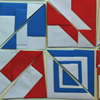### Paper Patchwork 2

##### Age 5 to 7 Challenge Level:

Have a go at making a few of these shapes from paper in different sizes. What patterns can you create?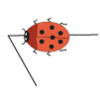### Walking Round a Triangle

##### Age 5 to 7 Challenge Level:

This ladybird is taking a walk round a triangle. Can you see how much he has turned when he gets back to where he started?### Data Shapes

##### Age 5 to 7 Challenge Level:

Sara and Will were sorting some pictures of shapes on cards. "I'll collect the circles," said Sara. "I'll take the red ones," answered Will. Can you see any cards they would both want?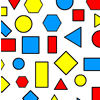### Sorting Logic Blocks

##### Age 5 to 11 Challenge Level:

This activity focuses on similarities and differences between shapes.### Jig Shapes

##### Age 5 to 11 Challenge Level:

Can you each work out what shape you have part of on your card? What will the rest of it look like?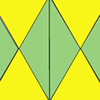### Repeating Patterns

##### Age 5 to 7 Challenge Level:

Try continuing these patterns made from triangles. Can you create your own repeating pattern?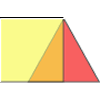### Overlaps

##### Age 5 to 7 Challenge Level:

What does the overlap of these two shapes look like? Try picturing it in your head and then use some cut-out shapes to test your prediction.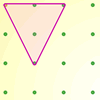### Inside Triangles

##### Age 5 to 7 Challenge Level:

How many different triangles can you draw on the dotty grid which each have one dot in the middle?### Matching Triangles

##### Age 5 to 7 Challenge Level:

Can you sort these triangles into three different families and explain how you did it?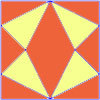### Fraction Fascination

##### Age 7 to 11 Challenge Level:

This problem challenges you to work out what fraction of the whole area of these pictures is taken up by various shapes.### More Transformations on a Pegboard

##### Age 7 to 11 Challenge Level:

Use the interactivity to find all the different right-angled triangles you can make by just moving one corner of the starting triangle.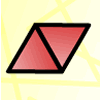### Triangle Animals

##### Age 5 to 7 Challenge Level:

How many different ways can you find to join three equilateral triangles together? Can you convince us that you have found them all?### Property Chart

##### Age 11 to 14 Challenge Level:

A game in which players take it in turns to try to draw quadrilaterals (or triangles) with particular properties. Is it possible to fill the game grid?### Shapely Pairs

##### Age 11 to 14 Challenge Level:

A game in which players take it in turns to turn up two cards. If they can draw a triangle which satisfies both properties they win the pair of cards. And a few challenging questions to follow...### Nine-pin Triangles

##### Age 7 to 11 Challenge Level:

How many different triangles can you make on a circular pegboard that has nine pegs?### Isosceles Triangles

##### Age 11 to 14 Challenge Level:

Draw some isosceles triangles with an area of $9$cm$^2$ and a vertex at (20,20). If all the vertices must have whole number coordinates, how many is it possible to draw?### Hexy-metry

##### Age 14 to 16 Challenge Level:

A hexagon, with sides alternately a and b units in length, is inscribed in a circle. How big is the radius of the circle?### Transformations on a Pegboard

##### Age 7 to 11 Challenge Level:

How would you move the bands on the pegboard to alter these shapes?### Rectangle Tangle

##### Age 7 to 11 Challenge Level:

The large rectangle is divided into a series of smaller quadrilaterals and triangles. Can you untangle what fractional part is represented by each of the shapes?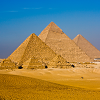### Egyptian Rope

##### Age 7 to 11 Challenge Level:

The ancient Egyptians were said to make right-angled triangles using a rope with twelve equal sections divided by knots. What other triangles could you make if you had a rope like this?### Lens Angle

##### Age 14 to 16 Challenge Level:

Find the missing angle between the two secants to the circle when the two angles at the centre subtended by the arcs created by the intersections of the secants and the circle are 50 and 120 degrees.### Cut it Out

##### Age 7 to 11 Challenge Level:

Can you dissect an equilateral triangle into 6 smaller ones? What number of smaller equilateral triangles is it NOT possible to dissect a larger equilateral triangle into?### Triangle Midpoints

##### Age 14 to 16 Challenge Level:

You are only given the three midpoints of the sides of a triangle. How can you construct the original triangle?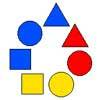### Chain of Changes

##### Age 5 to 7 Challenge Level:

Arrange the shapes in a line so that you change either colour or shape in the next piece along. Can you find several ways to start with a blue triangle and end with a red circle?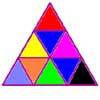### Colouring Triangles

##### Age 5 to 7 Challenge Level:

Explore ways of colouring this set of triangles. Can you make symmetrical patterns?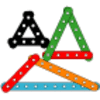### Let's Investigate Triangles

##### Age 5 to 7 Challenge Level:

Vincent and Tara are making triangles with the class construction set. They have a pile of strips of different lengths. How many different triangles can they make?### Bracelets

##### Age 7 to 11 Challenge Level:

Investigate the different shaped bracelets you could make from 18 different spherical beads. How do they compare if you use 24 beads?### Possible Pairs

##### Age 7 to 11 Challenge Level:

In this game, you turn over two cards and try to draw a triangle which has both properties.### Name That Triangle!

##### Age 7 to 11 Challenge Level:

Can you sketch triangles that fit in the cells in this grid? Which ones are impossible? How do you know?### Triangle or No Triangle?

##### Age 5 to 7 Challenge Level:

Here is a selection of different shapes. Can you work out which ones are triangles, and why?### Triangle Transformation

##### Age 7 to 14 Challenge Level:

Start with a triangle. Can you cut it up to make a rectangle?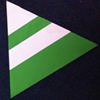### Regular Rings 1

##### Age 7 to 11 Challenge Level:

Can you work out what shape is made by folding in this way? Why not create some patterns using this shape but in different sizes?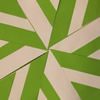### Folding Flowers 2

##### Age 7 to 11 Challenge Level:

Make a flower design using the same shape made out of different sizes of paper.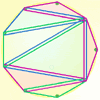### Board Block for Two

##### Age 5 to 7 Challenge Level:

Board Block game for two. Can you stop your partner from being able to make a shape on the board?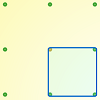### Peg and Pin Boards

##### Age 5 to 11

This article for teachers suggests activities based on pegboards, from pattern generation to finding all possible triangles, for example.### Constructing Triangles

##### Age 11 to 14 Challenge Level:

Generate three random numbers to determine the side lengths of a triangle. What triangles can you draw?### Calculating with Cosines

##### Age 14 to 18 Challenge Level:

If I tell you two sides of a right-angled triangle, you can easily work out the third. But what if the angle between the two sides is not a right angle?### Cutting it Out

##### Age 5 to 11 Challenge Level:

I cut this square into two different shapes. What can you say about the relationship between them?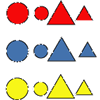### Logic Block Collections

##### Age 5 to 7 Challenge Level:

What do you think is the same about these two Logic Blocks? What others do you think go with them in the set?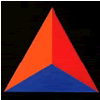### Animated Triangles

##### Age 5 to 7 Challenge Level:

Watch this "Notes on a Triangle" film. Can you recreate parts of the film using cut-out triangles?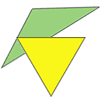### Triangle Relations

##### Age 7 to 11 Challenge Level:

What do these two triangles have in common? How are they related?### Putting Two and Two Together

##### Age 7 to 11 Challenge Level:

In how many ways can you fit two of these yellow triangles together? Can you predict the number of ways two blue triangles can be fitted together?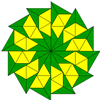### Triangle Shapes

##### Age 5 to 11 Challenge Level:

This practical problem challenges you to create shapes and patterns with two different types of triangle. You could even try overlapping them.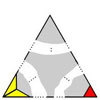### Impossible Triangles?

##### Age 16 to 18 Challenge Level:

Which of these triangular jigsaws are impossible to finish?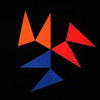### Notes on a Triangle

##### Age 11 to 14 Challenge Level:

Can you describe what happens in this film?### Lighting up Time

##### Age 7 to 14 Challenge Level:

A very mathematical light - what can you see?### The Square Hole

##### Age 14 to 16 Challenge Level:

If the yellow equilateral triangle is taken as the unit for area, what size is the hole ?### Equal Equilateral Triangles

##### Age 14 to 16 Challenge Level:

Can you make a regular hexagon from yellow triangles the same size as a regular hexagon made from green triangles ?### Interacting with the Geometry of the Circle

##### Age 5 to 16

Jennifer Piggott and Charlie Gilderdale describe a free interactive circular geoboard environment that can lead learners to pose mathematical questions.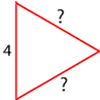### Number the Sides

##### Age 7 to 11 Challenge Level:

The triangles in these sets are similar - can you work out the lengths of the sides which have question marks?